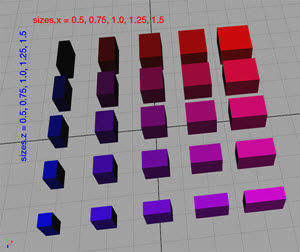# assetApproxRatio function

### Syntax

• string assetApproxRatio(searchQuery, axesRatio, n)

### Parameters

1. searchQuerystring
Search query to apply on list of all files in the workspace. See fileSearch / filesSearch for details about the syntax.
2. axesRatiostring
{ "xy" | "xz" | "yz" | "xyz" }—Axes for the current scope.
3. nfloat
Number (integer >= 1) of assets to consider (one is randomly picked out of the n best assets).

### Returns

Asset with one of the best n size fits (according to axesRatio).

### Description

The assetApproxRatio function returns one of the n best assets of the best ratio, from the files specified in searchQuery, according to the specified axesRatio string.

##### Note:

assetApproxRatio(searchQuery, axesRatio, 1) == assetBestRatio(searchQuery, axesRatio)

### Examples

#### Inserting assets based on their approximate ratio

 The goal is to insert assets from a pool, depending on their (physical) size ratio. The pool of assets is seen in the following image. Colors are (only) used to visually emphasize the physical size ratio.##### Note:
• Since the assets are color coded with their ratio, it is visible that "long" and "wide" Lots utilize black and pink assets, while "square-ish" Lots utilize red and blue assets.
• Note the color variations in the next two images.

#### n=1: "best ratio"

 ``````Lot --> innerRectangle(scope) { shape : Insert } Insert --> alignScopeToAxes(y) s('1, 0, '1) i(assetApproxRatio( "/myProject/assets/cube_*.obj", "xz", 1))``````#### n = 3: "choose randomly one of the best three ratios"

 ``````Lot --> innerRectangle(scope) { shape : Insert } Insert --> alignScopeToAxes(y) s('1, 0, '1) i(assetApproxRatio( "/myProject/assets/cube_*.obj", "xz", 3))``````